Documentation

### This is machine translation

Mouseover text to see original. Click the button below to return to the English version of the page.

## Model and Validate a System

You model each component within the system structure to represent the physical or functional behavior of that component. You verify the basic component behavior by simulating them using test data.

A big-picture view of the whole system layout is useful when modeling individual components. Start by loading the layout model:

```open_system(fullfile(matlabroot,... 'help', 'toolbox', 'simulink', 'examples', 'system_layout'))```

### Model the Components

A Simulink® model of a component is based on several starting points:

• An explicit mathematical relationship between the output and the input of a physical component — You can compute the outputs of the component from the inputs, directly or indirectly, through algebraic computations and integration of differential equations. For example, computation of the water level in a tank given the inflow rate is an explicit relationship. Each Simulink block executes based on the definition of the computations from its inputs to its outputs.

• An implicit mathematical relationship between model variables of a physical component — Because variables are interdependent, assigning an input and an output to the component is not straightforward. For example, the voltage at the + end of a motor connected in a circuit and the voltage at the – end have an implicit relationship. To model such a relationship in Simulink, you can either use physical modeling tools such as Simscape™ or model these variables as part of a larger component that allows input/output definition. Sometimes, closer inspection of modeling goals and component definitions helps to define input/output relationships.

• Data obtained from an actual system — You have measured input/output data from the actual component, but a fully defined mathematical relationship does not exist. Many devices have unmodeled components that fit this description. An example would be the heat a TV set dissipates. You can use System Identification Toolbox™ to define the input/output relationship for such a system.

• An explicit functional definition — You define the outputs of a functional component from the inputs through algebraic and logical computations. The switching logic of a thermostat is an example. You can model most functional relationships as Simulink blocks and subsystems.

This tutorial models physical and functional components with explicit input/output relationships:

1. Use the system equations to create a Simulink model.

2. Add Simulink blocks in the Simulink Editor. Blocks represent coefficients and variables from the equations. Connect blocks.

3. Build the model for each component separately. The most effective way to build a model of a system is to consider components independently.

4. Start by building simple models using approximations of the system. Identify assumptions that can affect the accuracy of your model. Iteratively add detail until the level of complexity satisfies the modeling and accuracy requirements.

#### Model the Physical Components

Describe the relationships between components, for example, data, energy, and force transfer. Use the system equations to build a graphical model of the system in Simulink.

Some questions to ask before you begin to model a component:

• What are the constants for each component and the values that do not change unless you change them?

• What are the variables for each component and the values that change over time?

• How many state variables does a component have?

Derive the equations for each component using scientific principles. Many system equations fall into three categories:

• For continuous systems, differential equations describe the rate of change for variables with the equations defined for all values of time. For example, a second-order differential equation provides the velocity of a car:

• For discrete systems, difference equations describe the rate of change for variables, but the equations are defined only at specific times. For example, the following difference equation gives the control signal from a discrete propositional-derivative controller:

• Equations without derivatives are algebraic equations. For example, an algebraic equation gives the total current in a parallel circuit with two components:

Wheels and Linear Motion.  There are two forces that act on a wheel:

• Force applied by the motor — This force F acts in the direction of velocity change, and is an input to the wheel subsystem.

• Drag force — This force Fdrag acts against the direction of velocity change, and is a function of the velocity itself:

`${F}_{drag}={k}_{drag}V|V|$`

The acceleration is proportional to the sum of these forces:Where kdrag is the drag coefficient and m is the mass of the robot. Each wheel carries half of this mass.

Build the wheel model:

1. In the layout model, double-click the Right Wheel subsystem to display the empty subsystem. Delete the connection between the Inport and the Outport blocks.

2. Model velocity and acceleration. Add an Integrator block. Leave the initial condition as `0`. The output of this block is the velocity, V, and the input is the acceleration, Vdot.

3. Model the drag force. Add an Fcn block from the User-Defined Functions library. Set the expression to `k_drag*u*abs(u)`. You can resize the block to see the expression on its icon. The Fcn block provides a quick way to type simple mathematical expressions of one input variable, u.

4. Subtract the drag force from the motor force with Subtract block, and complete the force-acceleration equation with a Gain block with parameter `1/(2*m)`.

5. To reverse the direction of the Fcn block, right-click the block and select Rotate & Flip > Flip Block. Make the connections between blocks as shown.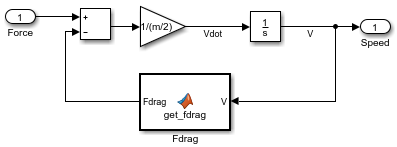6. View the top level of the model: Click the button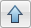. Make a copy of the subsystem you modeled as the dynamics for both wheels are the same.

Rotational Motion.  When the two wheels turn in opposite directions, that is, they have directionally opposite velocities, they move in a circle with radius r, causing rotational motion. When they turn in the same direction, there is no rotation. Therefore, with the assumption that the wheel velocities are always the same in magnitude, it is practical to model rotational motion as dependent on the difference of the two velocities, VR and VL:

`$\stackrel{˙}{\theta }=\frac{{V}_{R}-{V}_{L}}{2r}$`

Build the Rotation Dynamics model:

1. In the layout model, double-click the Rotation subsystem to display the empty subsystem. Delete the connection between the Inport and the Outport.

2. Model angular speed and angle: Add an Integrator block. Leave the initial condition as `0`. The output of this block is the angle, theta, and the input is the angular speed, theta_dot.

3. Compute angular speed from tangential speed. Add a Gain with parameter `1/(2*r)`.

4. Make the connections between blocks as follows.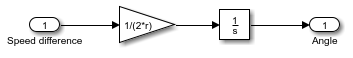5. View the top level: Click the button.

#### Model the Functional Components

Describe the function from the input of a function to its output. This description can include algebraic equations and logical constructs, which you can use to build a graphical model of the system in Simulink.

Coordinate Transformation.  The velocity of the robot in the X and Y coordinates, Vx and Vy, are related to the linear speed, Vn, and the angle as follows:

`$\begin{array}{l}{V}_{X}=-{V}_{N}\mathrm{cos}\left(\theta \right)\\ {V}_{Y}={V}_{N}\mathrm{sin}\left(\theta \right)\end{array}$`

Build coordinate transformation model:

1. In the layout model, double-click the Coordinate Transform subsystem to display the empty subsystem.

2. Model trigonometric functions. Add a SinCos block from the Math Operations library.

3. Model multiplications. Add two Product blocks from the Math Operations library.

4. Make connections between the blocks as shown.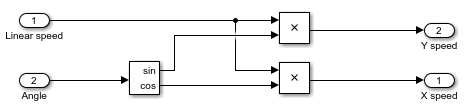5. View the top level: Click the button.

#### Set Model Parameters

A source for model parameter values can be:

• Written specifications such as standard property tables or manufacturer data sheets

• Direct measurements on an existing system

• Estimations using system input/output

The model uses these parameters:

ParameterSymbolValue/Unit
Massm2.5 kg
Rolling resistancek_drag30 Ns2/m

Simulink uses MATLAB® workspace to evaluate parameters. Set these parameters in the MATLAB command window:

```m = 2.5; k_drag = 30; r = 0.15;```

### Validate Components Using Simulation

Validate components by supplying an input and observing the output. Even such a simple validation can point out immediate ways to improve the model. This example validates the following behavior:

• When a force is applied continuously to a wheel, the velocity increases until it reaches a steady-state velocity.

• When the wheels are turning in opposite directions, the angle increases steadily.

#### Validate Wheel Component

Create and run a test model for the wheel component:

1. Create a model. Click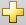and copy the Right Wheel block into the new model.

2. Create a test input in the new model. Add a Step block from the Sources library. Connect it to the input of the Right Wheel block.

3. Add a viewer to the output. Right-click the output port of the Right Wheel block and select Create & Connect Viewer > Simulink > Scope.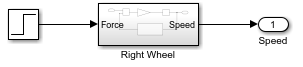4. Run the simulation. Click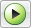.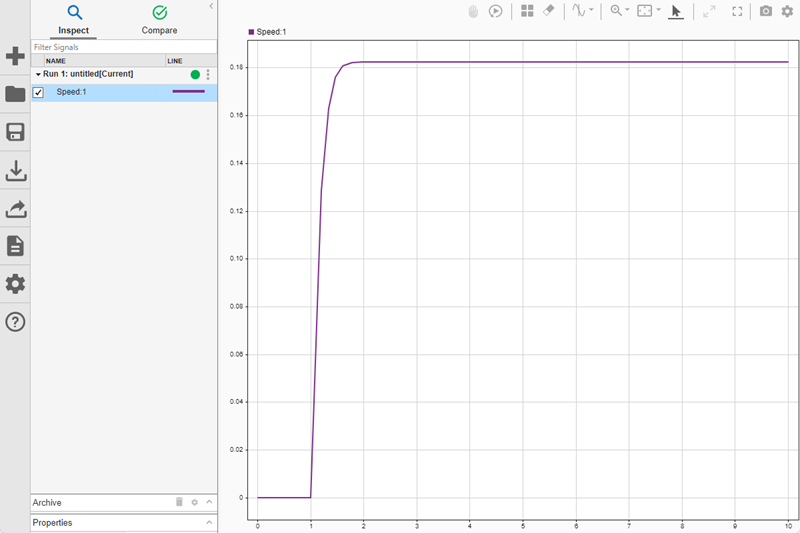The simulation result exhibits the general expected behavior. There is no motion until force is applied at step time. When force is applied, the speed starts increasing and then settles at a constant when the applied force and the drag force reach an equilibrium. Besides validation, this simulation also gives information on the maximum speed of the wheel with the given force.

#### Validate Rotation Component

Create and run a test model for the rotation model:

1. Create a model. Clickand copy the Rotation block into the new model.

2. Create a test input in the new model. Add a Step block from the Sources library. Connect it to the input of the Rotation block. This input represents the difference of the wheel velocities when the wheels are rotating in opposite directions.

3. Add a viewer to the output. Right-click the output port of the Rotation block and select Create & Connect Viewer > Simulink > Scope.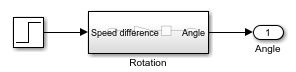4. Run the simulation. Click.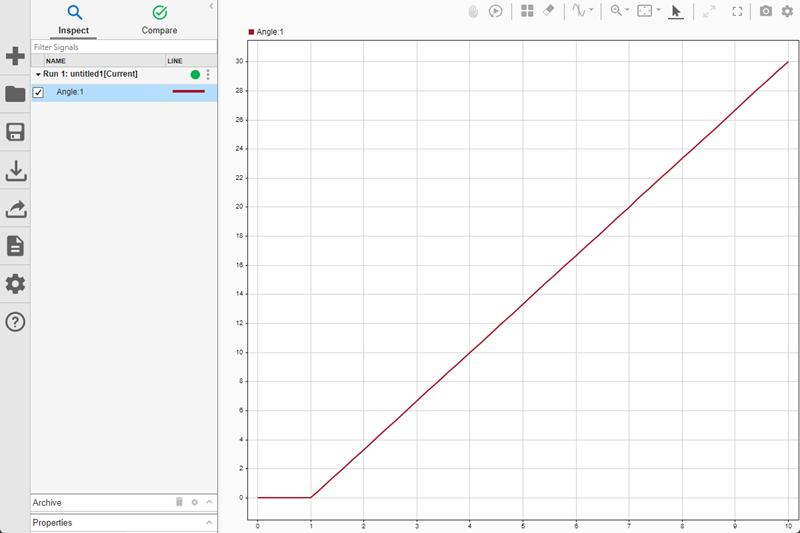This simulation shows that the angle increases steadily when the wheels are turning with the same speed in opposite directions. You can make some model improvements to make it easier to interpret the angle output, for example:

• You can convert the output in radians to degrees. Add a Gain block with a gain of `180/pi`.

• You can display the degrees output in cycles of 360 degrees. Add a Math Function block with function `mod`.

MATLAB trigonometric functions take inputs in radians.

### Validate the Model

After you validate components, you can perform a similar validation on the complete model. This example validates the following behavior:

• When the same force is applied to both wheels in the same direction, the vehicle moves in a line.

• When the same force is applied to both wheels in opposite directions, the vehicle turns around itself.

1. In the layout model, double-click the Inputs subsystem to display the empty subsystem.

2. Create a test input by adding a Step block. Connect it to both Outport blocks.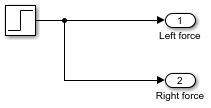3. At the top level of the model, add both output signals to the same viewer: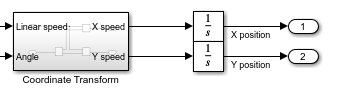4. Run the model.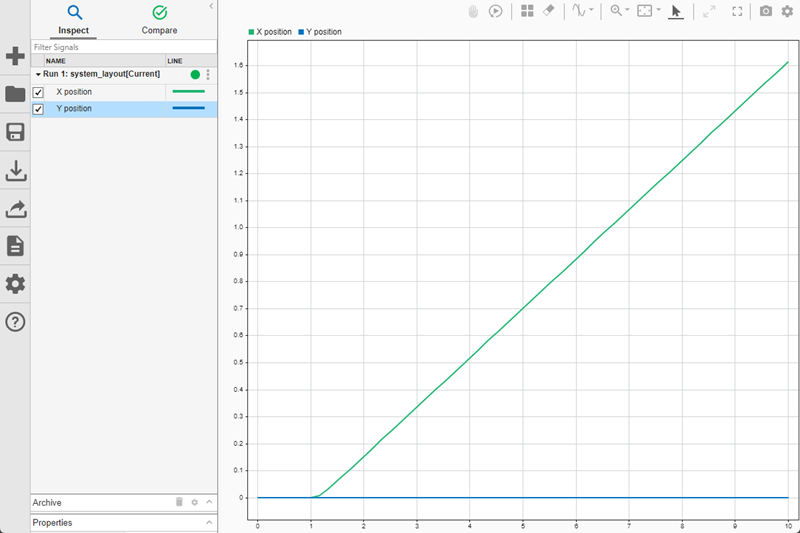In this figure, the yellow line is the X direction and the blue line is the Y direction. Since the angle is zero and is not changing, the vehicle moves only in the X direction, as expected.

5. Double-click the Inputs subsystem and add a Gain with parameter `-1` between the source and the second output. This reverses the direction for the left wheel.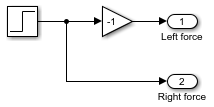6. Add a scope to the angle output.

7. Run the model.The first view shows that there is no motion in the X-Y plane. The second view shows that there is steady rotation.

You can use this final model to answer many questions about the model by changing the input. Some examples are:

• What happens when the initial angle is not zero?

• How long does it take for the motion to stop when the force drops to zero?

• What happens when the robot is heavier?

• What happens when the robot moves on a smoother surface, that is, the drag coefficient is lower?